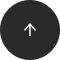Standard SQL

# How to Round Numbers in SQL

ROUND

#### Problem:

You want to round a number to a specific number of decimal places.

#### Example:

Our database has a table named `product` with data in the following columns: `id`, `name`, and `price_net`.

idnameprice_net
2croissant1.22
3roll0.68

Suppose there’s a tax of 24% on each product, and you’d like to compute the gross price of each item (i.e., after taxes) and round the value to two decimal places.

#### Solution:

```SELECT id, ROUND( price_net*1.24 , 2 ) as price_gross
FROM product;
```

This query returns the gross price rounded to two decimal places:

idprice_gross
12.90
21.51
30.84

#### Discussion:

If you’d like to round a floating-point number to a specific number of decimal places in SQL, use the ROUND function. The first argument of this function is the column whose values you want to round; the second argument is optional and denotes the number of places to which you want to round. By default, if you don’t specify the second argument, the function rounds to the nearest integer.

In this example, we won’t specify the number of places to which we want to round the column:

```SELECT id, ROUND(price_net*1.24) as price_gross
FROM product;
```

And here’s the corresponding result:

idprice_gross
13
22
31

#### Recommended articles: